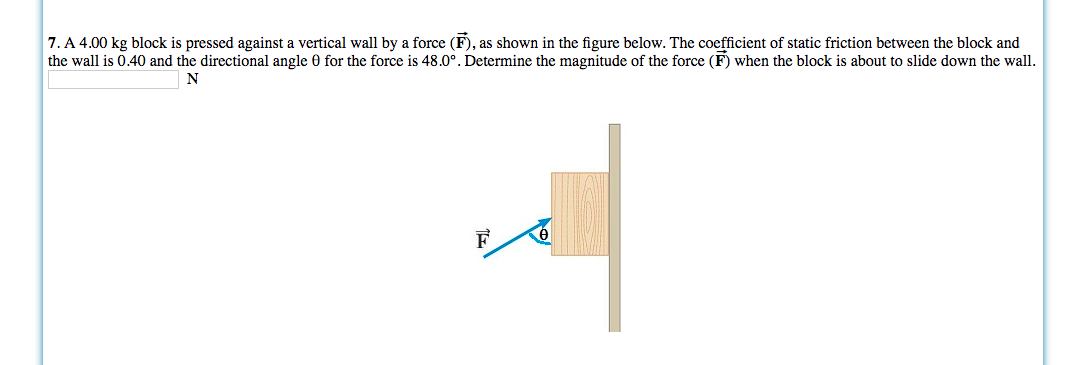# 7. A 4.00 kg block is pressed against a vertical wall by a force (F), as shown in the figure below. The coefficient of static friction between the block and the wall is 0.40 and the directional angle 0 for the force is 48.0°. Determine the magnitude of the force (F) when the block is about to slide down the wall F

Question

A 4.00 kg block is pressed against a vertical wall by a force (→F), as shown in the figure below. The coefficient of static friction between the block and the wall is 0.40 and the directional angle θ for the force is 48.0°. Determine the magnitude of the force (→F) when the block is about to slide down the wall.help_outlineImage Transcriptionclose7. A 4.00 kg block is pressed against a vertical wall by a force (F), as shown in the figure below. The coefficient of static friction between the block and the wall is 0.40 and the directional angle 0 for the force is 48.0°. Determine the magnitude of the force (F) when the block is about to slide down the wall F fullscreen

### Want to see the step-by-step answer?